# It's all regression

We almost always work with variables that are either …

• quantitative
• categorical with only two levels

In relating two variables, there are four possible situations

Quantitative Categorical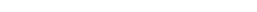Quant. slope of regression line or correlation coefficient difference in two means
…………. …………………………………… ……………………………………….
Categ. ?????? difference in two proportions

## Presentation in terms of algebra

Quantitative CategoricalQuant. $$b_1 = \frac{n\sum xy - \sum x \cdot \sum y}{n \sum x^2 - (\sum x)^2}$$ $$t = \frac{\bar{x}_1 - \bar{x}_2 - (\mu_1 - \mu_2)}{\sqrt{\frac{s_p^2}{n_1} + \frac{s_p^2}{n_2}}}$$
…………. …………………………………… ……………………………………….
Categ. ?????? $$z = \frac{(\hat{p_1} - \hat{p_2}) - (p_1 - p_2)}{\sqrt{\frac{\bar{p}\bar{q}}{n_1} + \frac{\bar{p}\bar{q}}{n_2}}}$$

… and associated distributions: t and z

## Data beats formulas

Quantitative CategoricalQuant.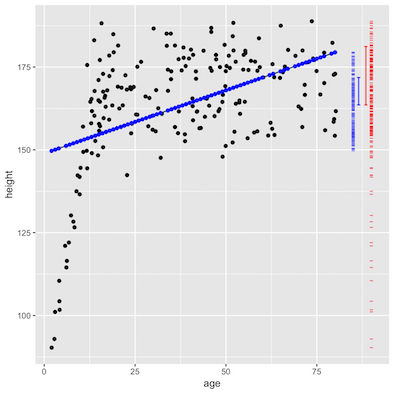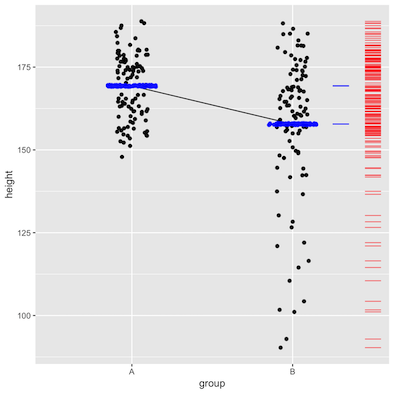…………. …………………………………… ……………………………………….
Categ.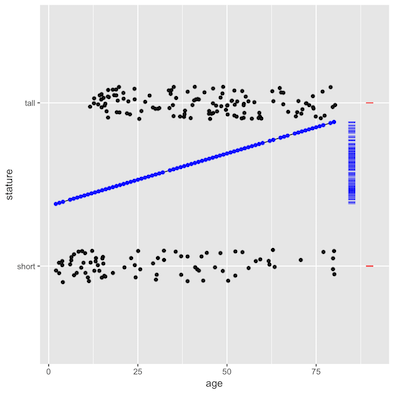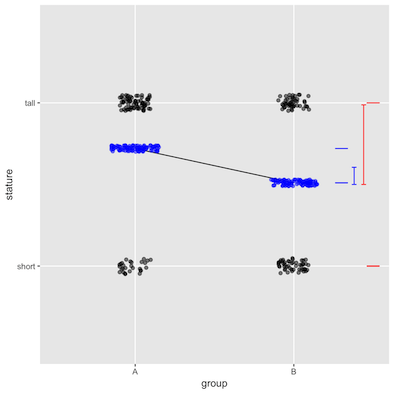## Raw and model values

The error bars show the size of the standard deviation.

knitr::include_graphics("/images/PQQ.png")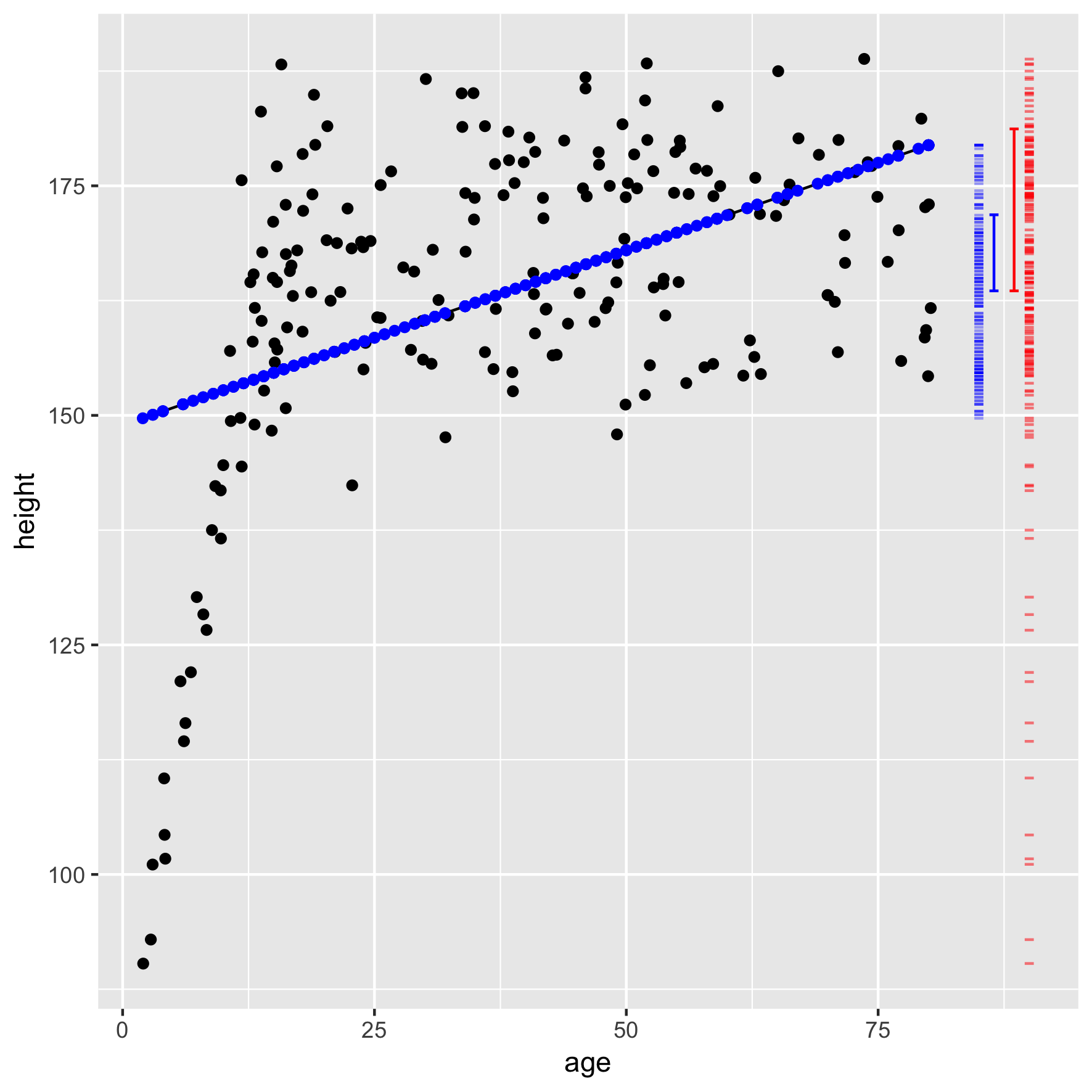## The formal inference procedure

1. Measure the effect size. Call it $$\beta$$:
• slope for Quant vs Quant or Cat vs Quant
• difference for Quant vs Cat or Cat vs Cat
2. Take the ratio of the model-value standard deviation to the raw-value standard deviation. Call this ratio $$R$$.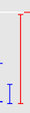1. Compute the ratio of “explained” to “unexplained”: $F = (n-1) \frac{R^2}{1 - R^2}$

Eyeballing from the four models (which had n = 200)…

Quantitative Categorical
Quantitative $$\beta = \frac{1\ cm}{3\ years}$$           $$R \approx 0.5$$ $$F = 24.8$$ $$\beta \approx 8\ cm$$,        $$R \approx 1/3$$ $$F \approx 66$$

Categorical $$\beta = 0.007$$ per year,   $$R \approx 0.12$$       $$F \approx 8.3$$ $$\beta \approx 0.2$$,        $$R \approx 0.25$$       $$F \approx 13.3$$

1. Confidence interval on $$\beta$$ is always $CI_\beta = \beta (1 \pm 2 / \sqrt{F})$

2. p-value … Look up in this graph.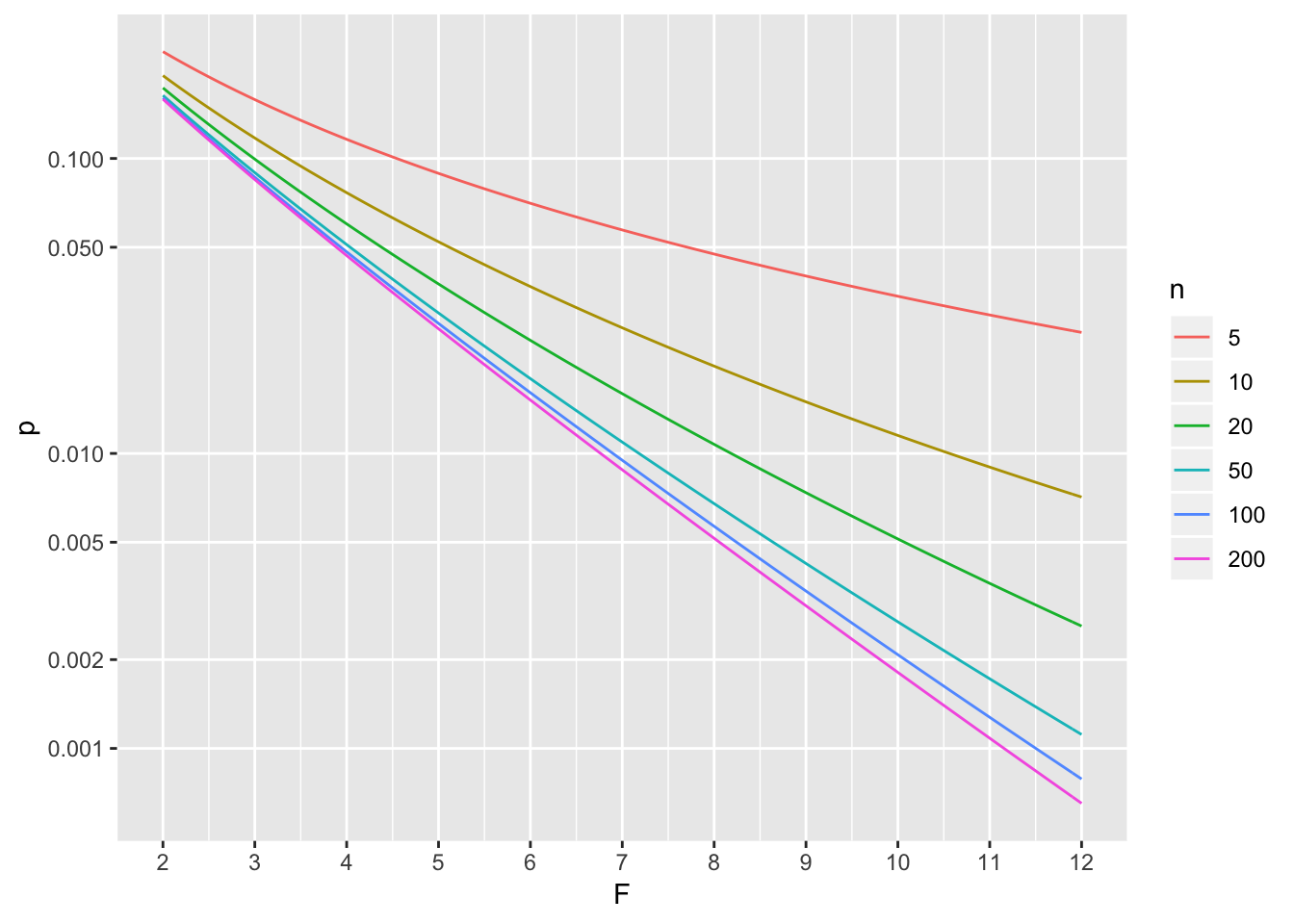Freebies:

• correlation coefficient $$r = \sqrt{F}$$, where the $$\pm$$ branch is based on the slope.
• Prefer t? It’s $$t = \sqrt{F}$$. But F is more general than t.